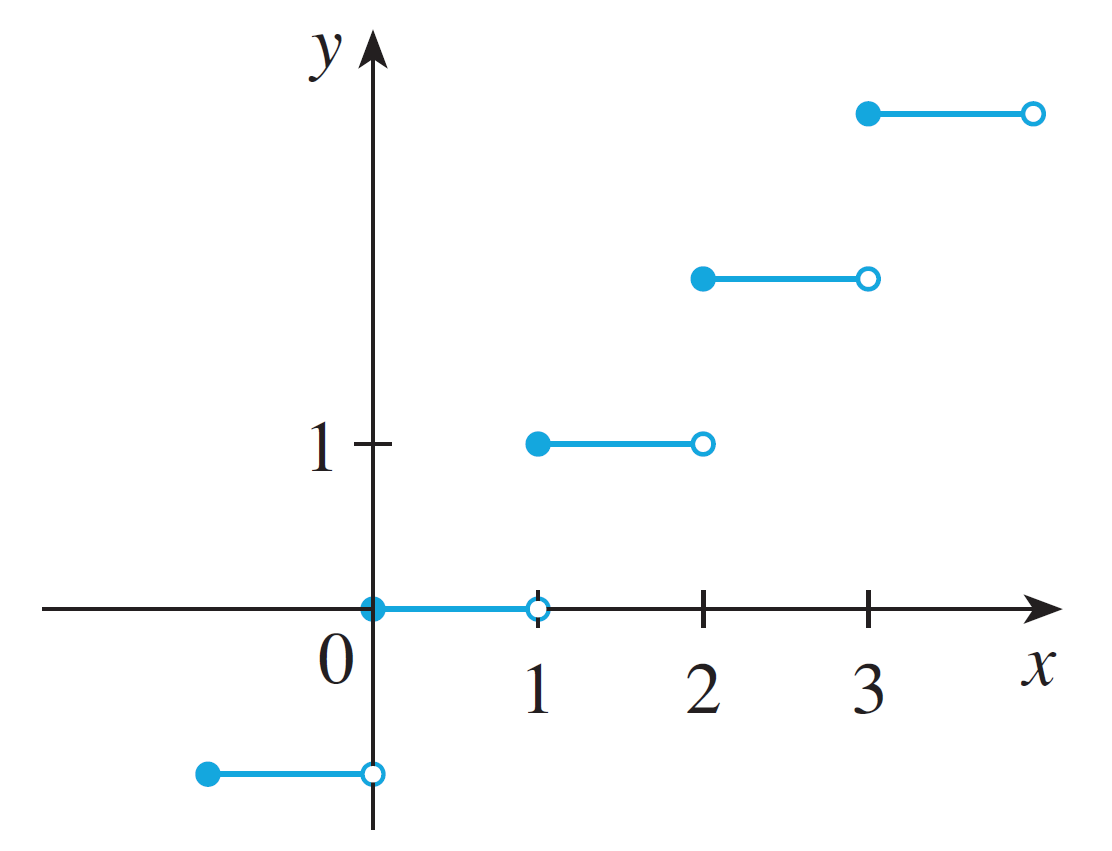# Homework 3

Directions: Show each step of your work. Turn in your answers in class on a physical piece of paper. Staple multiple sheets together. Feel free to use Desmos for graphing.

1. Find the following limits with the appropriate technique.
1. $\displaystyle \lim_{x\rightarrow 1}\dfrac{\sqrt{x} - 1}{x - 1}$
2. $\displaystyle \lim_{x\rightarrow 0}\dfrac{2x^2 - 2x}{x}$
3. $\displaystyle \lim_{x\rightarrow -2}\dfrac{x^2 - 4}{x^3 + 2x^2}$
4. $\displaystyle \lim_{x\rightarrow \infty}\dfrac{x^4 + x^2 + 1}{x^3 + x + 3}$
5. $\displaystyle \lim_{x\rightarrow \infty}\dfrac{x^3 + 2x^2 - x - 6}{3x^3 -2x^2 + 4x}$
6. $\displaystyle \lim_{x\rightarrow \infty}\dfrac{x^2 + 1}{x^4 - x}$
2. It is estimated that the total population of San Luis Obispo is given by the function $P(t) = \dfrac{25t^2 + 125t + 200}{t^2 + 5t + 20}$ where $t$ is years since $2020$.
Evaluate $\displaystyle\lim_{t\rightarrow \infty} P(t)$ and interpret the meaning of the limit in English.
3. Given this graph of $f(x)$Determine which statements are true or false.
1. $\displaystyle\lim_{x\rightarrow 0^-} f(x) = 0$
2. $\displaystyle\lim_{x\rightarrow 0^+} f(x) = 0$
3. $f(1)$ is defined.
4. $\displaystyle\lim_{x\rightarrow 2} f(x) = f(2)$
5. $\displaystyle\lim_{x\rightarrow 1} f(x)= 1$
6. $\displaystyle\lim_{x\rightarrow 3^-} f(x)= \lim_{x\rightarrow 3^+}f(x)$
4. Suppose a function $f(x)$ has $f(2) = 5$ and $\displaystyle \lim_{x \rightarrow 2}f(x) = 5$. Is the function continuous at $x = 2$?
5. Given each function, use the definition of continuity to determine where the function is discontinuous.
1. $\displaystyle f(x) = \dfrac{2}{2x - 1}$
2. $f(x) = x^{100} + 2x^{99} + 95x^5 + 99x^2 + 1$
3. $\displaystyle f(x) = \begin{cases}x & x \leq 1 \\ 2x - 1 & x > 1\end{cases}$
4. $\displaystyle f(x) = \begin{cases}x + 5 & x < 0 \\ 2 & x = 0 \\ -x^2 + 5 & x > 0\end{cases}$
6. Draw the graph of a function which is continuous on $(-\infty, -2) \cup (-2, 3) \cup (3, \infty)$ and $\displaystyle\lim_{x\rightarrow 3}f(x)$ does not exist.
7. Suppose $f(x) = 2x^{12324538742389}$. Are we allowed to use the limit laws to find $\displaystyle \lim_{x\rightarrow 3^+} f(x)$?
8. Suppose $f(x)$ is a function. What are the two meanings of $f'(x)$?
9. Suppose I have a function $f(x)$. If $f'(5)$ doesn't exist, state all the possibilities why it does not.
10. Suppose I have a function $f(x)$. When is $f(x)$ differentiable at $x = 5$? Hint: definition.
11. Bank of America has undergone a series of bad loans in agriculture and real estate. The losses are estimated to be (in millions of dollars) $f(t) = -t^2 + 10t + 30$ where $t$ is the time in years since 2020.
1. What is the rate of change of the losses in 2021? Use the definition, not shortcuts.
12. When we are using the definition of the derivative:
1. What always happens to the $h$ in the denominator?
2. Suppose the above phenomena does not happen. What is the problem?
13. For the following functions, use the definition of the derivative. Find the following:
1. Find the instantaneous rate of change of $f(x) = 5x$ at $x = 3$.
2. $\displaystyle \dfrac{d}{dx} (x^2 - 4x)$
3. If $f(x) = 3x^2$, find $f'(x)$.
14. Suppose $f(x)$ is differentiable on $\mathbb{R}$. Must $f(x)$ be continuous on $\mathbb{R}$? If not, explain why.
15. Suppose $f(x)$ is continuous on $\mathbb{R}$. Must $f(x)$ be differentiable on $\mathbb{R}$? If not, draw the graph of a function where it fails to be differentiable.
16. Suppose I have $f'(x) = \lim_{h\rightarrow 0} \dfrac{\sqrt{9 + h} - 3}{h}$What is $f(x)$?